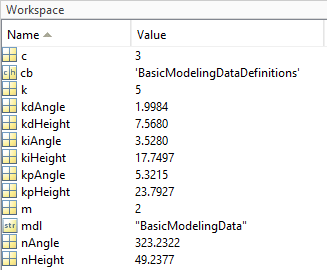# Manage Model Data

To define a parameter value that multiple blocks or models use, you can use variables. Separately updating numerical parameter values at each instance of the parameter can be inefficient and error prone. You can update the value of a variable in the workspace or source file that defines it. Having a single source for this information facilitates scalability and reusability of model components.

To specify value ranges, data types, tunability, and other characteristics of signals, states, and block parameters, you can use the `Simulink.Parameter` and `Simulink.Signal` objects. While you can use variables or objects to specify parameter values, this example uses variables for simplicity.

You can define variables by using these supporting file types:

• MAT-file

• MATLAB file

• Data dictionary

To load data for small models, you can use model callbacks. For large model hierarchies, different loading methods are more efficient.

In model `ex_modeling_data`, a `PreLoadFcn` model callback evaluates MATLAB file `ex_modeling_data_variables.m`, which defines variables,, andin the base workspace. Gain blocks in the mechanical system and PID Controller blocks in the controller use these variables.You can interactively change variable values at the MATLAB® command prompt. You can also use tools like the Model Data Editor to edit values.